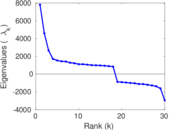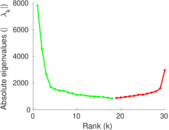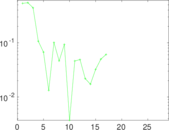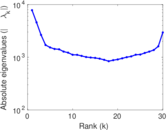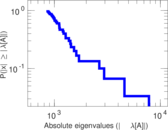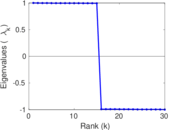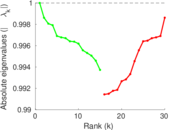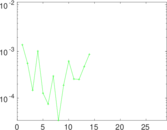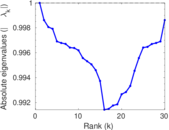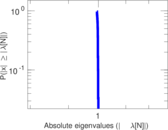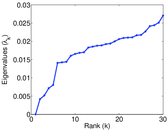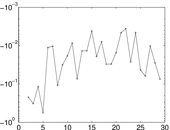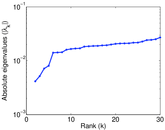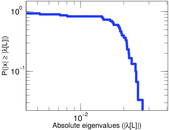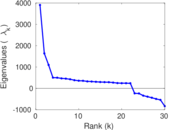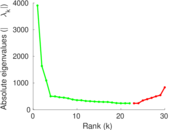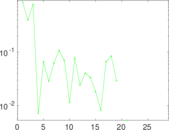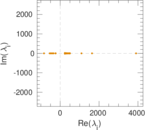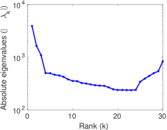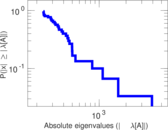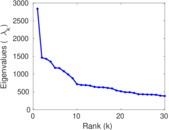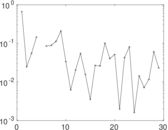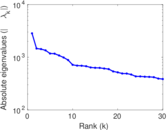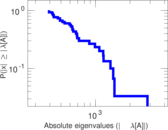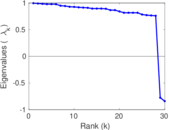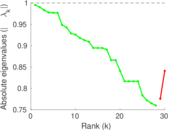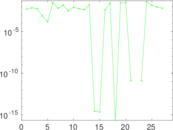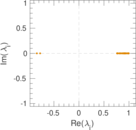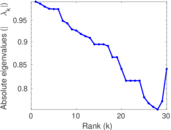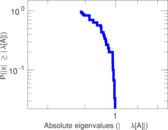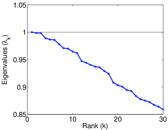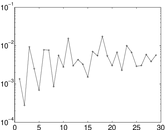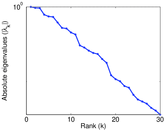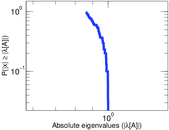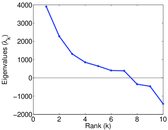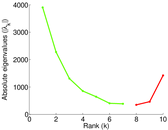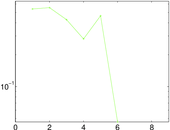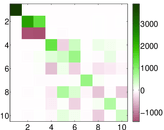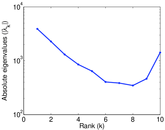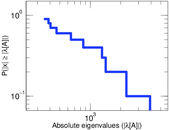# Enron

The Enron email network consists of 1,148,072 emails sent between employees of Enron between 1999 and 2003. Nodes in the network are individual employees and edges are individual emails. It is possible to send an email to oneself, and thus this network contains loops.

 Code `EN` Internal name `enron` Name Enron Data source http://www.cs.cmu.edu/~enron/ AvailabilityDataset is available for download Consistency checkDataset passed all tests Category Communication network Dataset timestamp 1999 ⋯ 2003 Node meaning Employee Edge meaning Email Network format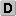Unipartite, directed Edge typeUnweighted, multiple edges Temporal dataEdges are annotated with timestamps ReciprocalContains reciprocal edges Directed cyclesContains directed cycles Loops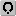Contains loops

## Statistics

 Size n = 87,273 Volume m = 1,148,072 Unique edge count m̿ = 321,918 Wedge count s = 49,424,399 Claw count z = 13,894,959,120 Cross count x = 3,805,295,597,320 Triangle count t = 1,180,387 Square count q = 92,985,807 4-Tour count T4 = 942,178,964 Maximum degree dmax = 38,785 Maximum outdegree d+max = 32,619 Maximum indegree d−max = 6,166 Average degree d = 26.309 9 Fill p = 4.226 54 × 10−5 Average edge multiplicity m̃ = 3.566 35 Size of LCC N = 84,384 Size of LSCC Ns = 9,164 Relative size of LSCC Nrs = 0.105 004 Diameter δ = 14 50-Percentile effective diameter δ0.5 = 4.405 46 90-Percentile effective diameter δ0.9 = 5.790 34 Median distance δM = 5 Mean distance δm = 4.903 26 Balanced inequality ratio P = 0.100 618 Outdegree balanced inequality ratio P+ = 0.106 510 Indegree balanced inequality ratio P− = 0.134 857 Power law exponent γ = 2.651 60 Tail power law exponent γt = 1.761 00 Tail power law exponent with p γ3 = 1.761 00 p-value p = 0.000 00 Outdegree tail power law exponent with p γ3,o = 2.301 00 Outdegree p-value po = 0.000 00 Indegree tail power law exponent with p γ3,i = 2.871 00 Indegree p-value pi = 0.351 000 Degree assortativity ρ = −0.167 768 Degree assortativity p-value pρ = 0.000 00 In/outdegree correlation ρ± = +0.403 110 Clustering coefficient c = 0.071 648 0 Spectral norm α = 7,808.15 Operator 2-norm ν = 3,904.14 Cyclic eigenvalue π = 3,904.00 Algebraic connectivity a = 0.004 157 70 Reciprocity y = 0.146 497 Non-bipartivity bA = 0.622 292 Spectral bipartite frustration bK = 8.928 20 × 10−5 Controllability C = 77,596 Relative controllability Cr = 0.889 118

## Plots

### Degree distribution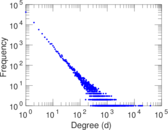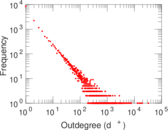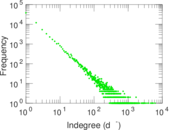### Cumulative degree distribution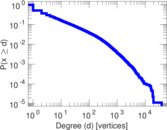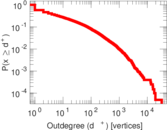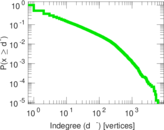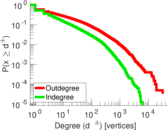### Lorenz curve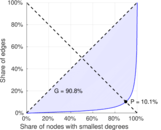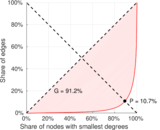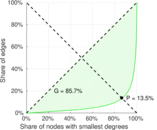### Spectral distribution of the adjacency matrix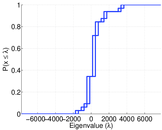### Spectral distribution of the normalized adjacency matrix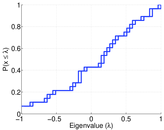### Spectral distribution of the Laplacian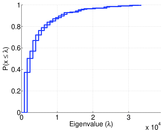### Spectral graph drawing based on the adjacency matrix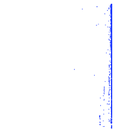### Spectral graph drawing based on the Laplacian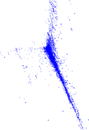### Spectral graph drawing based on the normalized adjacency matrix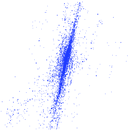### Degree assortativity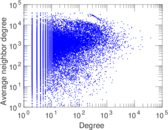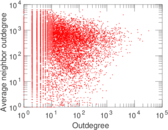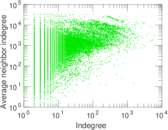### Zipf plot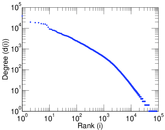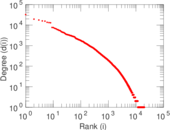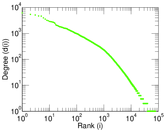### Hop distribution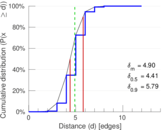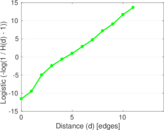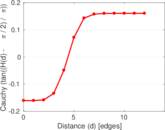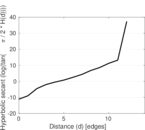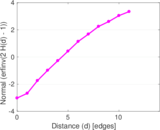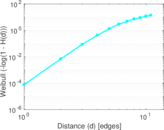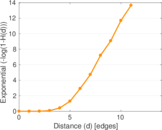### Delaunay graph drawing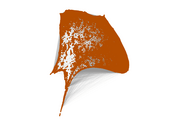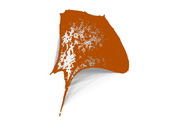### In/outdegree scatter plot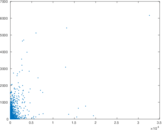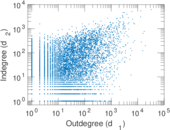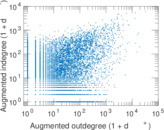### Edge weight/multiplicity distribution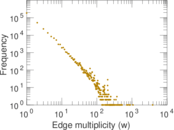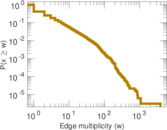### Clustering coefficient distribution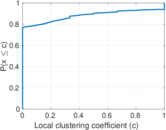### Temporal distribution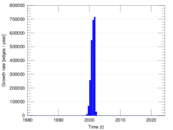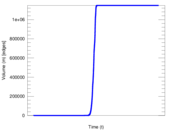### Temporal hop distribution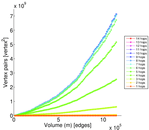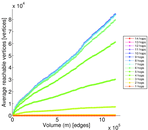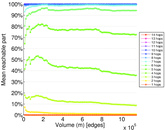### Diameter/density evolution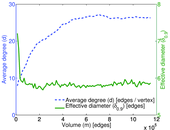### SynGraphy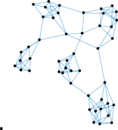### Inter-event distribution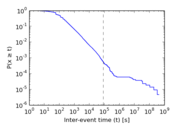### Node-level inter-event distribution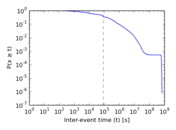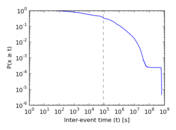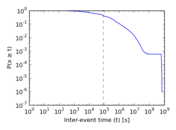### Matrix decompositions plots